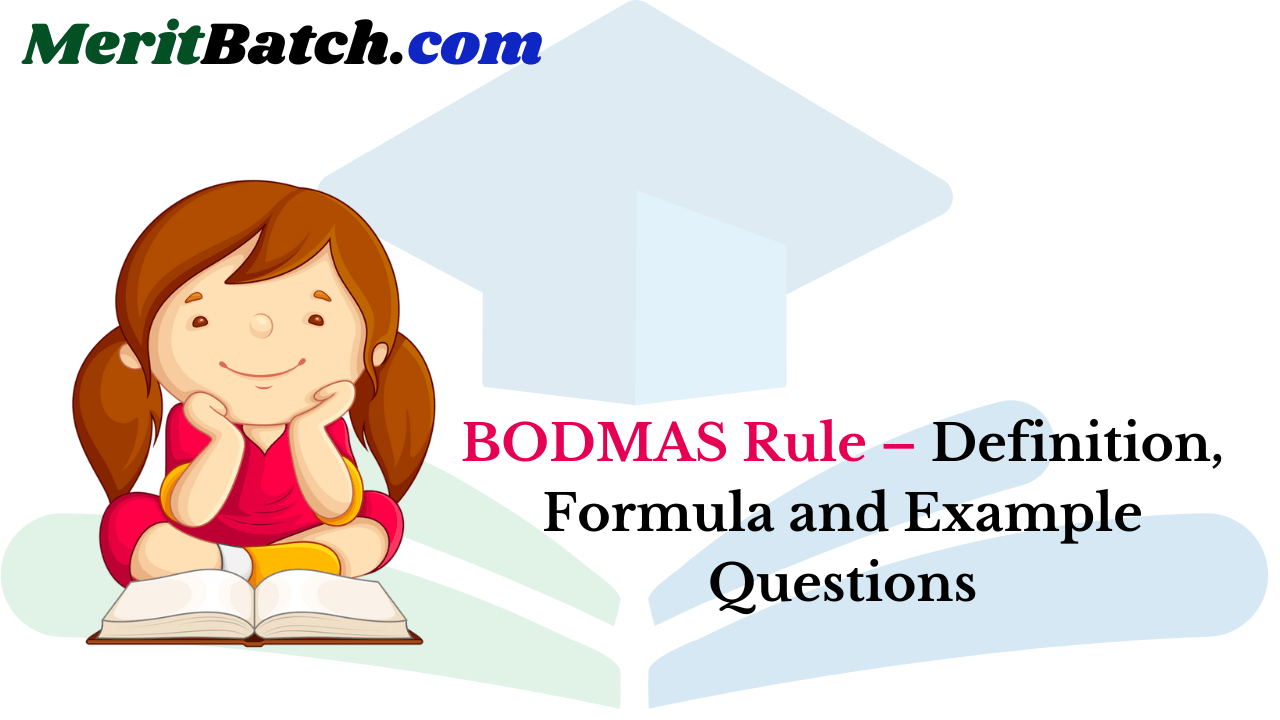## BODMAS RULE:

We must remember the word VBODMAS in solving sums on simplification. These letters stand for vinculum, bracket, of, division, multiplication, addition and subtraction respectively.

The sums on simplification must be solved in that order i.e., first solve vinculum followed by bracket and so on until the sum is solved.

 V Vinculum means bar as (-) B Bracket- () {} and then [ ] O of D Division [÷] M Multiplication [x] A Addition [+] S Subtraction [-]

In simplifying an expression first of all bar must be removed. After removing the bar, the brackets must be removed, strictly in the order ( ), { }and [ ].

After removing the brackets, we must use the following operations strictly in the order: (i) of (ii) Division (iii) Multiplication (iv) Addition (v) Subtraction

 Example 1 Example 2 B Brackets 5 × ( 7 – 3) = 5 × 4 = 20 24 ÷ ( 8 – 5 ) = 24 ÷ 3 = 8 O Orders 7 + 22 = 7 + 4 = 11 10 – 32 = 10 – 9 = 1 D Divide 9 + 12 ÷ 3 = 9 + 4 = 13 10 – 6 ÷ 2 = 10 – 3 = 7 M Multiply 10 ÷ 2× 3 = 10 + 6 = 16 9 – 4 × 2 = 9 – 8 = 1 A Add 4 × 3 + 5 = 12 + 5 = 17 2 × 7 + 8 = 14 + 8 = 22 S Subtract 10 ÷ 2 – 2 = 5 – 2 = 3 9 ÷ 3 – 1 = 3 – 1 = 2

Ordering Mathematical Operations

 B O D M A S Brackets [ ], { }, ( ) Orders x2, √x Divide ÷ Multiply × Addition + Subtract ×

## Use of brackets and the BODMAS rule

Let us consider an example to illustrate the use of brackets.
Rima bought 35 chocolates and ate 5 of them. She distributed the remaining chocolates equally among 6 of her friends. How many chocolates did she give to each of them?

In this problem we have to subtract 5 chocolates that Rima ate, from 35 chocolates she had, before dividing them among 6 of her friends. So we have to first perform the operation of subtraction and then do division. In such cases, we use brackets around the part that has to be done first, that is(35 – 5) ÷ 6 (First solve bracket, i.e., 35 – 5 = 30)
= 30 ÷ 6      (Division: 30 ÷ 6 = 5)
= 5
Consider another example.

Example 1: Solve 2 of 3 × (5 + 2).
Solution: 2 of 3 × (5 + 2)
= 2 of 3 × 7  (First bracket: 5 + 2 = 7)
= 6 × 7          (Of: 2 of 3 = 2 × 3 = 6)
= 42              (Multiplication: 6 × 7 = 42)
Hence, when problems involve brackets, of, ×, ÷, +, and – thenTo make it easy to remember this order, we remember the word BODMAS, where B stands for brackets, O for of’, D for division, M for multiplication, A for addition, and S for subtraction. This is called the ‘BODMAS‘ rule.
Sometimes numerical expressions may involve different types of brackets. These brackets are

• Vinculum or bar —
• Parentheses or small brackets ( )
• Braces or curly brackets { }
• Square brackets or big brackets [ ]

We simplify expressions by starting with the innermost bracket. Usually the vinculum is the innermost bracket, next is the parentheses, then the braces, and finally the square brackets. Let us now consider some examples.

Example 2: Simplify $$25-[20-\{10-(7-\overline{5-3})\}]$$.
Solution:Example 3: Simplify [72 -12 ÷ 3 of 2] + (18 – 6) ÷ 4.
Solution:RULES FOR SIMPLIFICATION

1. Order of operation: The use of brackets take us to a new order of operation. The operation inside the brackets comes before the ODMAS. There are different types of brackets already mentioned here.

2. If there is no sign between a number and the bracket, then it is implied that the operation to be performed is multiplication.
Examples3. When there is ‘+’ sign before a bracket, you can simply remove the bracket.

Examples:4. When there is a ‘–’ sign before a bracket, then all signs within the bracket change while removing the bracket.

Examples:Ex: 1 :
Simplify:Solution:Exercise
1.2.3.4.1.  c     2. a      3. d      4. c

2. Application of the formula, (a + b)2 = a2 + b2 + 2ab
Ex: 1.
Simplify 0.46 x 0.46 + 0.54 x 0.54 + 0.92 x 0.54
Solution:
We have the expression
0. 46 x 0.46 + 0.54 x 0.54 + 0.92 x 0.54
(0.46)2 +(0.54)2 + 2. x 0.46 x 0.54
If we suppose a = 0.46 and b = 0.54, then
= a2 + b2 + 2ab = (a + b)2
= (0.46 + 0.54)2 = (1.00)2 = 1